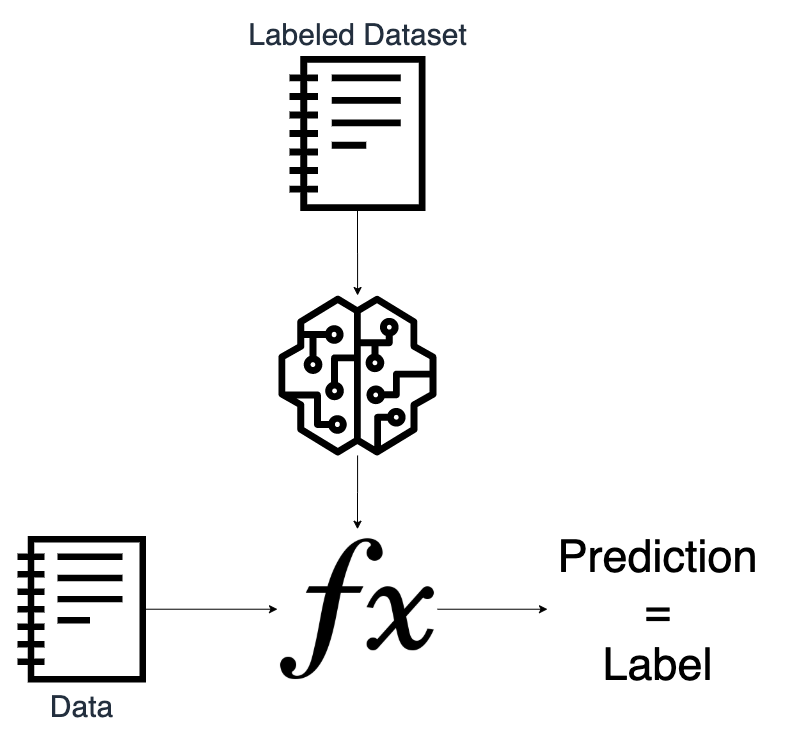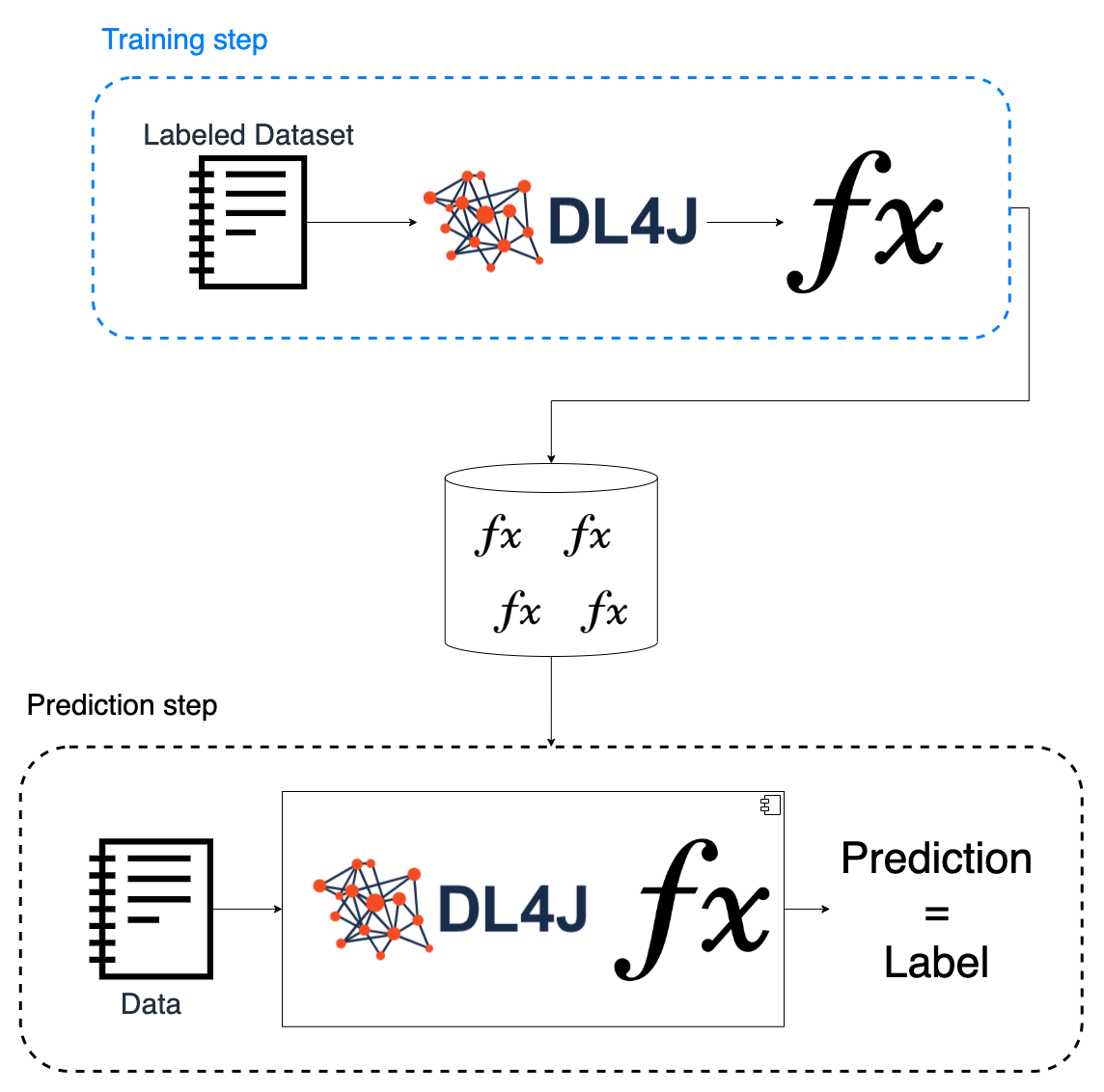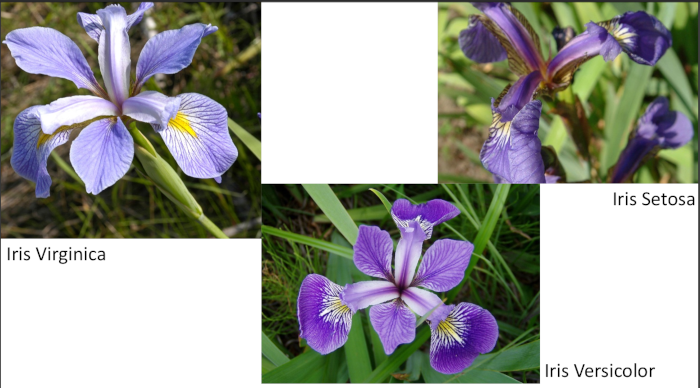# TL; DR

• Machine Learning is the study of computer algorithms that improve automatically through experience .
• Deeplearning4j is an Open-source deep-learning library written for Java, Scala, Kotlin, and Clojure.
• The Machine Learning “hello world”, the iris dataset, is used to explain the basics of Deeplearning4j.
• The goal of this post is to train and export a model. The prediction step is covered in the next post.
• All the code for this example can be found at the repository deeplearning4j-playground, more specifically at the file IrisClassifierTrainer.java.

# Machine Learning in 130 words

There are different types of Machine Learning, e.g. supervised, unsupervised, reinforcement learning. This post will focus on supervised learning.

The goal of supervised Machine Learning is to extrapolate or “predict” data, either a label or a number. This is done with mathematical functions, which were found looking for relationships on a training data set. Having said this, we can identify two main steps: the training step and the prediction step.

The input of the training step is a labeled data set, i.e. columns of data with a label. Its output is a mathematical function aka model capable to map a new set of data to a known label. The input of the prediction step is the model previously generated and a new set of data. Its output is a label.Basic steps of Machine Learning. Image by the author of this post.

# Deeplearning4j: what is?

Deeplearning4j is an Open-source deep-learning library written for Java, Scala, Kotlin, and Clojure. In an ecosystem where Python has the biggest chunk, Deeplearning4j enables the people with mostly Java knowledge to work with machine learning, also exploiting the strengths of the Java ecosystem, like its maturity and production readiness.

### Supported approaches

The basic classification of machine learning approaches are :

• Supervised: A data set containing features and labels are fed into a machine learning algorithm to find rules that map the data and the labels. There are 2 basic subtypes: – Classification: The features are mapped to a label or class – Regression: The features are mapped to a number.
• Unsupervised: A data set without labels are fed into a machine learning algorithm to find relationships amongst the data, like hidden patterns, or to find a structure.
• Reinforcement learning: An agent interacts with an environment using a set of actions looking to maximize a reward.

If you want to know more, have a look at Machine Learning: Approaches

Deeplearning4j supports all three of the basic machine learning approaches.

### Supported algorithms

There are a lot of different kinds of algorithms in machine learning, for example:

• Support Vector Machines
• Linear regression
• Logistic regression
• Naive Bayes
• Linear discriminant analysis
• Decision trees
• Neural Networks (Multilayer perceptron)

The Neural Networks are the basis for the broadly known deep learning. As its name indicates, Deeplearning4j focuses on neural networks and is the only kind of algorithm it supports.

# Hands on example

We will see how to use Deeplearning4j applying it to the Iris Classification Dataset, AKA the Machine Learning Hello World. In this post, we will focus on the training step. The prediction step will come in the next post of the series.Training and prediction steps used in Machine Learning. Image by the author of this post.

## Prerequisites

1. A Java development environment
2. A dependency manager. Maven is used in this example.
3. The Iris data set. It is all over the internet, e.g. on the Wikipedia page Iris flower data set.

## Steps

1. Dataset description
2. Problem description
3. Dependencies to include
4. Load the dataset
5. Normalize the data
6. Split in train and test datasets
7. Configure the model
8. Train the model
9. Evaluate the model
10. Export the model
11. Automated testing

### 1. Dataset description

The Iris data set “The data set consists of 50 samples from each of three species of Iris (Iris Setosa, Iris Virginica, and Iris Versicolor). Four features were measured from each sample: the length and the width of the sepals and petals, in centimeters.”  Based on these features, we want to find a model capable of classifying a species. More information can be found on the wikipedia page.The three species of Iris (Iris setosa, Iris virginica and Iris versicolor). Source: https://en.wikipedia.org/wiki/Iris_flower_data_set

### 2. Problem description

• Type: Classification
• Dataset:
• Number of instances: 150
• Number of attributes/features: 4
• Sepal length in cm
• Sepal width in cm
• Petal length in cm
• Petal width in cm
• Number of classes/labels: 3
• Iris Setosa
• Iris Versicolour
• Iris Virginica

### 3. Dependencies to include

In this post, I will assume the usage of Maven to handle dependencies. Also, no graphic card optimization will be used.

`````` <!-- https://mvnrepository.com/artifact/org.deeplearning4j/deeplearning4j-core -->
<dependency>
<groupId>org.deeplearning4j</groupId>
<artifactId>deeplearning4j-core</artifactId>
<version>\${dl4j.version}</version>
</dependency>

<!-- https://mvnrepository.com/artifact/org.nd4j/nd4j-native-platform -->
<dependency>
<groupId>org.nd4j</groupId>
<artifactId>nd4j-native-platform</artifactId>
<version>\${dl4j.version}</version>
</dependency>
``````

For a full example of the pom.xml, visit deeplearning4j-examples: pom.xml

### 4. Load the dataset

The goal of this step is to load into memory the data set from the disk. In this case, as it is a small data set, we just load all of it directly.

In this case, we use a `CSVRecordReader` because that is the format of the data set.

After that, on the DataSetIterator, we indicate:

• How many lines we want to read per iteration (in this case `TOTAL_LINES`, as we will load all the data set directly),
• What is the index where the label is (`LABEL_INDEX`)
• And how many different classes are available (`CLASSES_COUNT = 3`, the 3 types of Iris flowers)
``````private static DataSet loadData(String path) throws IOException,
InterruptedException {
DataSet allData;
TOTAL_LINES, LABEL_INDEX, CLASSES_COUNT);
allData = iterator.next();
}
return allData;
}
``````

Once the data is loaded, we need to shuffle it. Failing to do this will cause the model to perform badly.

``````DataSet allData = loadData(datasetPath);
allData.shuffle(SEED);
``````

### 5. Normalize the data

The idea of normalization is making the data fit always in the range of [0,1] or [-1,1], so that big numbers in certain features will not have a bigger effect on small numbers in other features. Of course, this has pros and cons. If you want to read more about normalization, have a look at Feature_scaling or Understand Data Normalization in Machine Learning .

In this case, we use the `NormalizerStandardize`, which as per the documentation “will standardize and de-standardize data arrays, based on statistics of the means and standard deviations of the population”.

``````DataNormalization normalizer = new NormalizerStandardize();
// Get stats about the data
normalizer.fit(allData);
// Transform the data by applying the normalization
normalizer.transform(allData);
``````

### 6. Split in train and test data sets

The goal of this step is to get two subsets of the data to use them with different purposes:

1. Train data set: This subset is used to train the model.
2. Test data set: This subset is used to evaluate the model. The idea is to feed the model with data it does not know about to determine how accurate its predictions are.

Fortunately, we can use a built-in method to do this. We need to pass a parameter to indicate how many rows of the data set will be dedicated for training and how many will be for evaluation. In this case, it will be 65% for training.

``````private static final double TRAIN_TO_TEST_RATIO = 0.65;
...
// Split in train and test datasets
SplitTestAndTrain testAndTrain = allData.splitTestAndTrain(TRAIN_TO_TEST_RATIO);
DataSet trainingData = testAndTrain.getTrain();
DataSet testData = testAndTrain.getTest();
``````

### 7. Configure the model

As previously stated, Deeplearning4j supports only Neural Networks. In this case, we will use a neural network with 3 layers. Each layer has input nodes and output nodes and the amount of them can vary.

The most important layers are the input and the output layers:

• Input layer: it must have the same amount of input nodes as features on the data set: 4 (Sepal length, Sepal width, Petal length, Petal width)
• Output layer: it must have the same amount of output nodes as classes/labels on the dataset: 3 (Iris Setosa, Iris Versicolour, Iris Virginica)
``````private static final int CLASSES_COUNT = 3;
private static final int FEATURES_COUNT = 4;
...
private static MultiLayerConfiguration getMultiLayerConfiguration() {
return new NeuralNetConfiguration.Builder()
.seed(SEED)
.activation(Activation.TANH)
.weightInit(WeightInit.XAVIER)
.updater(new Sgd(0.1))
.l2(1e-4)
.list()
// The input layer must have FEATURES_COUNT = 4 nodes
.layer(new DenseLayer.Builder().nIn(FEATURES_COUNT).nOut(3)
.build())
.layer(new DenseLayer.Builder().nIn(3).nOut(3)
.build())
// The output layer must have CLASSES_COUNT = 3 nodes
.layer( new OutputLayer.Builder(
LossFunctions.LossFunction.NEGATIVELOGLIKELIHOOD)
.activation(Activation.SOFTMAX)
.nIn(3).nOut(CLASSES_COUNT).build())
.build();
}
``````

### 8. Train the model

Once we retrieve the configuration of the model, we instantiate it and initialize it. We can also set a listener, e.g. to be able to see the score every X parameter updates.

After that, we call the method `fit` of the `model` providing the training data set as input. It is a matter of experimentation to find out the right amount of training iterations to achieve the desired performance.

``````// Get configuration of the Neural Network
MultiLayerConfiguration configuration = getMultiLayerConfiguration();

// Train Neural Network
MultiLayerNetwork model = new MultiLayerNetwork(configuration);
model.init();
//Print score every 100 parameter updates
model.setListeners(new ScoreIterationListener(100));

// Do TRAIN_ITERATIONS = 1000 iterations to train the model
for(int x = 0; x < TRAIN_ITERATIONS; x++) {
model.fit(trainingData);
}
``````

### 9. Evaluate the model

In this step, we use the test data set to evaluate the performance of the model. For data, we feed all the test data set to the model and use its result as input for the `Evaluation` class provided by the library.

``````private static Evaluation evaluate(MultiLayerNetwork model, DataSet testData) {
INDArray output = model.output(testData.getFeatures());
Evaluation eval = new Evaluation(CLASSES_COUNT);
eval.eval(testData.getLabels(), output);
log.info(evaluation.stats());
return eval;
}
``````

The console output of this looks like:

``````========================Evaluation Metrics========================
# of classes:    3
Accuracy:        0.9245
Precision:       0.9206
Recall:          0.9167
F1 Score:        0.9163
Precision, recall & F1: macro-averaged (equally weighted avg. of 3 classes)

=========================Confusion Matrix=========================
0  1  2
----------
21  0  0 | 0 = 0
0 15  1 | 1 = 1
0  3 13 | 2 = 2

Confusion matrix format: Actual (rowClass) predicted as (columnClass) N times
==================================================================
``````

If you want to know more about all these metrics, you can check Accuracy, Precision, Recall or F1?.

### 10. Export the model

To store the model we use the `save` method it has, providing the path to store it and a boolean indicating whether to save the updater or not. As per the documentation “If true: save the updater (i.e., the state array for momentum/Adam/rmsprop etc), which should usually be saved if further training is required”

One important aspect here is that we need to also store the normalizer because we will need it later on in the prediction step. It is required then because we need to transform the input data the same way it was transformed before the training so that when we feed it to the model, the quantities make sense. Another way to see this is: the model was trained with values between 0 and 1, so if we feed it with values outside that range, e.g. 12.4, it will not be able to perform a proper prediction.

``````private void store(MultiLayerNetwork model,
DataNormalization normalizer,
String outputPath) throws IOException {
// Creating the folder to store the data
File baseLocationToSaveModel = new File(outputPath);
baseLocationToSaveModel.mkdirs();

// Storing the model
File locationToSaveModel = new File(outputPath + STORED_MODEL_FILENAME);
model.save(locationToSaveModel, false);

// Storing the normalizer
File locationToSaveNormalizer = new File(outputPath +
STORED_NORMALIZER_FILENAME);
NormalizerSerializer.getDefault().write(normalizer,
locationToSaveNormalizer);
log.info("Model and normalizer stored at {}", outputPath);
}
``````

### 11. Automated testing

Testing this is relatively easy: We just need to instantiate the `IrisClassifierTrainer` class (which contains all the functions that we have seen so far), and call the `train` function with the path to the data set. This method will export the trained model and return an evaluation object, which we can later assert with the criteria that we consider acceptable.

``````// Arbitrary number ;)
private static double MIN_ACCEPTABLE_ACCURACY = 0.90;

@Test
void testTrain() {
String irisDataset = "iris.txt";
String irisDatasetPath = getClass().getClassLoader()
.getResource(irisDataset).getPath();

String outputPath = "models/iris_classification/";
IrisClassifierTrainer irisClassifierTrainer =
new IrisClassifierTrainer(outputPath);
Evaluation evaluation = irisClassifierTrainer.train(irisDatasetPath,
"unit_test");

assertTrue(evaluation.accuracy() > MIN_ACCEPTABLE_ACCURACY);
}

``````

# Conclusion

At the end of this post, we saw how to train and export a model, so that in the next post, it will be picked up to perform predictions.

Furthermore, we covered:

• A minimalistic definition of machine learning
• Basic scope of Deeplearning4j
• What dependencies are required to use Deeplearning4j

And related to the hands-on example, the Iris data set, we saw:

• Dataset description
• Problem description
• How to load the dataset
• How to normalize the data
• How to split in train and test datasets
• How to configure the model
• How to train the model
• How to evaluate the model
• How to export the model
• How to do automated testing to train and assert the evaluation criteria of model

All the code for this example can be found at the repository deeplearning4j-playground, more specifically at the file IrisClassifierTrainer.java

I would love to see your tweets with feedback!

 Mitchell, T. (1997), Machine Learning. http://www.cs.cmu.edu/~tom/mlbook.html , retrieved 08/2020

 Machine Learning, Wikpedia. https://en.wikipedia.org/wiki/Machine_learning , retrieved 05/2021

 Deep_learning, Wikipedia. https://en.wikipedia.org/wiki/Deep_learning , retrieved 05/2021

 Iris flower data set, Wikipedia. https://en.wikipedia.org/wiki/Iris_flower_data_set , retrieved 05/2021